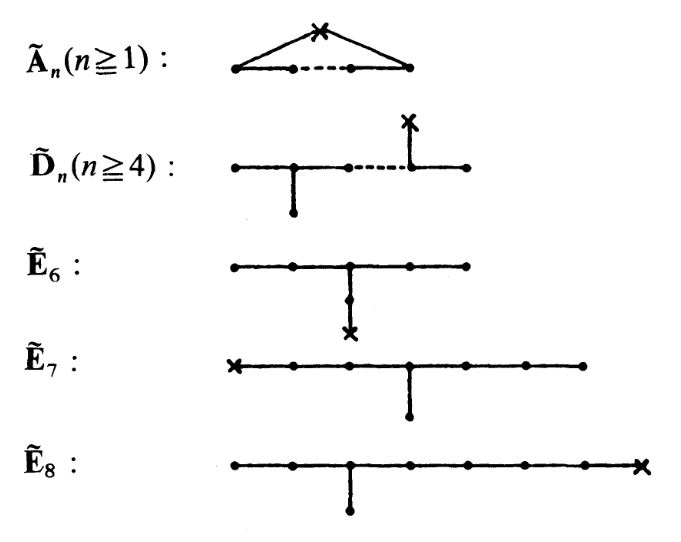nLab McKay quiver

Context

Representation theory

representation theory

geometric representation theory

Theorems

Graph theory

graph theory

graph

category of simple graphs

Contents

Idea

Generally, for $G$ a finite group and $V$ a linear representation of $G$ on a finite dimensional complex vector space, the McKay quiver or McKay graph associated with $V$ is the quiver whose vertices correspond to the irreducible representations $\rho_i$ of $G$ and which has $a_{i j} \in \mathbb{N}$ edges between the $i$th and the $j$th vertex, for $a_{i j}$ the coefficients in the expansion into irreps of the tensor product of representations of $V$ with these irreps:

$V \otimes \rho_i \;\simeq\; \underset{j}{\bigoplus} a_{i j} \cdot \rho_j \,.$

Specifically this applies to the special case where $G \subset$ SU(2) a finite subgroup of SU(2) and $V$ its defining representation on $\mathbb{C}^2$. The McKay correspondence states that in this case the corresponding McKay quivers are Dynkin quivers/Dynkin diagrams in the same ADE classification as the ADE singularity $\mathbb{C}^2 \sslash G$.

More precisely: If one uses all irreducible representations including the 1-dimensiona trivial representation $\rho_0$ then one gets the “extended Dynkin diagram”, where the extra node corresponds to $\rho_0$. This is the vertex indicated by a cross in the following diagrams:graphics grabbed from GSV 83, p. 4

In particular, for $G =\mathbb{Z}_N \subset SU(2)$ a cyclic group of order $N$, there are $N$ complex irreps and the McKay quiver, i.e. the extended Dynkin diagram, has $N$-vertices, connected by edges to form a circle.

The construction is due to

• John McKay, Graphs, singularities, and finite groups Proc. Symp. Pure Math. Vol. 37. No. 183. 1980

Interpretation in terms of equivariant K-theory of the corresponding ADE-singularity and plain K-theory of its blowup is due to